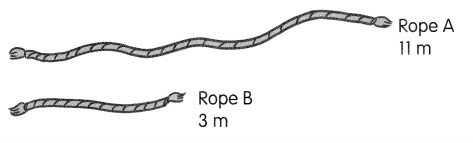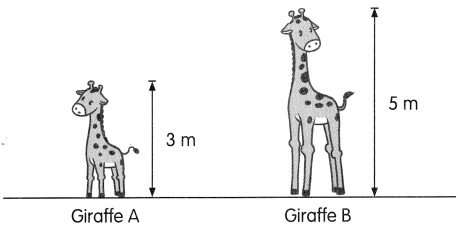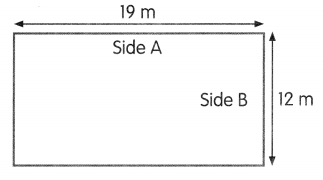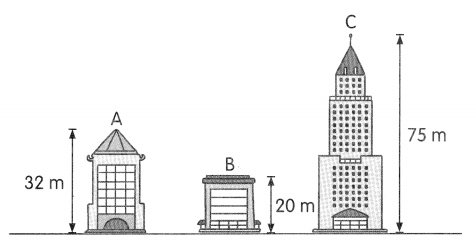# Math in Focus Grade 2 Chapter 7 Practice 2 Answer Key Comparing Lengths in Meters

Practice the problems of Math in Focus Grade 2 Workbook Answer Key Chapter 7 Practice 2 Comparing Lengths in Meters to score better marks in the exam.

## Math in Focus Grade 2 Chapter 7 Practice 2 Answer Key Comparing Lengths in Meters

Fill in the blanks.

Question 1.
Look at the two ropes.a. Which rope is longer? Rope ___
Answer: Rope A is longer than Rope B.
Explanation:
Given,
Rope A is 11 meters,
Rope B is 3 meters,
Therefore, Rope A is longer than Rope B.

b. How much longer is it? ___ m
Answer: Rope A is 8 meters longer than Rope B.
Explanation:
Given,
Rope A is 11 meters,
Rope B is 3 meters,
By subtracting 3 from 11 we get 8,
Therefore, Rope Ais 8 meters longer than Rope B.

Question 2.
Look at the two giraffes.a. Which giraffe is taller? Giraffe ___
Explanation:
Given,
Giraffe A is 3 meters long,
Giraffe B is 5 meters long,
Therefore, Giraffe B is taller than Giraffe A.

b. How much taller is it? ___ m
Answer: Giraffe B is 2 meters taller than Giraffe A.
Explanation:
Given,
Giraffe A is 3 meters long,
Giraffe B is 5 meters long,
By subtracting 3 from 5 we get 2,
Therefore, Giraffe B is 2 meters taller than Giraffe A

Question 3.
Look at the sides of the rectangle.a. Which is shorter, Side A or Side B? Side _____
Answer: Side is B is shorter than Side A
Explanation:
Given,
Side A is 19 meters,
Side B is 12 meters,
Therefore, Side B is shorter than Side A.

b. How much shorter is it? _____ m
Answer: Side B is 7 meters short.
Explanation:
Given,
Side A is 19 meters,
Side B is 12 meters,
By subtracting 12 from 19 we get 7,
Therefore, Side B is 7 meters shorter than Side A

Question 4.
Look at the buildingsa. Which building is the shortest? Building ____
Answer: Building B is the shortest with 20 meters height.

b. Which building is the tallest? Building ____
Answer: Building C is the tallest with 75 meters height.

c. Building B is ___ meters shorter than Building C.
Answer: Building B is 55 meters shorter than Building C.
Explanation:
By subtracting 20 from 75 we get 55,
Therefore, Building B is 55 meters shorter than Building C.

d. How much taller is Building C than Building A? ___ m
Answer: Building C is 43 meters taller than Building A.
Explanation:
By subtracting 32 from 75 we get 43,
Therefore, Building C is 43 meters taller than Building A.